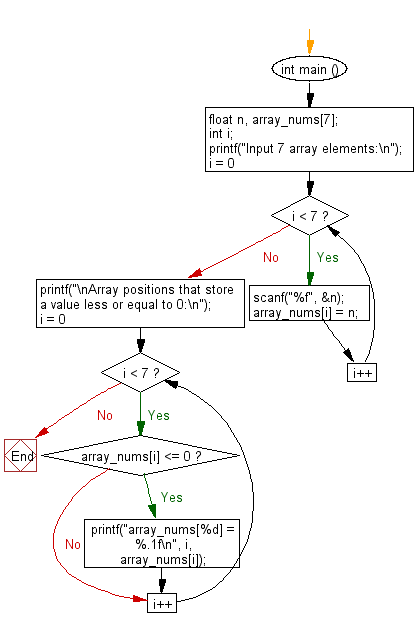﻿ C Program: Select specific array values - w3resource

# C Exercises: Select specific array values

## C Basic Declarations and Expressions: Exercise-126 with Solution

Write a C program that reads an array (length 7) and prints all array positions that store a value less than or equal to 0.

Sample Solution:

C Code:

``````#include <stdio.h>

int main () {
float n, array_nums;
int i;

// Prompt user for input
printf("Input 7 array elements:\n");

// Loop to read 7 float values from the user and store them in the array
for (i = 0; i < 7; i++) {
scanf("%f", &n);
array_nums[i] = n;
}

// Print a message indicating the positions with values less than or equal to 0
printf("\nArray positions that store a value less or equal to 0:\n");

// Loop to check and print positions with values less than or equal to 0
for (i = 0; i < 7; i++) {
if (array_nums[i] <= 0) {
printf("array_nums[%d] = %.1f\n", i, array_nums[i]);
}
}

return 0; // End of program
}
``````

Sample Output:

```Input 7 array elements:
15
23
37
65
20
-7
65

Array positions that store a value less or equal to 0:
array_nums = -7.0
```

Flowchart:C programming Code Editor:

What is the difficulty level of this exercise?

Test your Programming skills with w3resource's quiz.

﻿